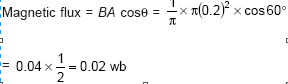Question 1

Which two of the following five physical parameters have the same dimensions? (a) energy density (b) refractive index (c) dielectric constant (d) Young's modulus (e) magnetic field

A : (a) and (e)
B : (b) and (d)
C : (c) and (e)
D : (a) and (d)

•
.

Solution :
Energy density & the Young's modulus have same dimensional formula.

•   Question 2

If the error in the measurement of radius of a sphere is 2%, then the error in the determination of volume of the sphere will be

A : 2%
B : 4%
C : 6%
D : 8%

•
.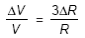•   Question 3

The distance travelled by a particle starting from rest and moving with an acceleration 4/3 ms-2 in the third second is

A : 19/3 m
B : 6m
C : 4m
D : 10/3 m

•
.

Solution :
Snth = u + a/2(2n-1)

•   Question 4

A particle moves in a straight line with a constant acceleration. It changes its velocity from 10 ms-1 to 20 ms-1 while passing through a distance 135 m in t second. The value of t is

A : 9
B : 10
C : 1.8
D : 12

•
.

Solution :
S = 1/2(u+v)t

•   Question 5

A particle shows distance-time curve as given in this figure. The maximum instantaneous velocity of the particle is around the point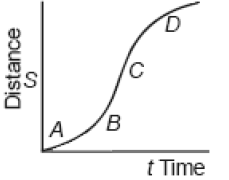A : A
B : B
C : C
D : D

•
.

Solution :
Maximum slope is at C.

•   Question 6

A particle of mass m is projected with velocity v making an angle of 45o with the horizontal. When the particle lands on the level ground the magnitude of the change in its momentum will be

A : 0
B : 2mv
C :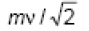D :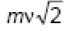•
.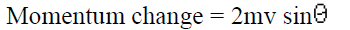•   Question 7

Sand is being dropped on a conveyor belt at the rate of M kg/s. The force necessary to keep the belt moving with a constant velocity of v m/s will be

A : 0
B : mv newton
C : 2mv newton
D : mv/2 newton

•
.

Solution :
Force= v(du|dt)=mv

•   Question 8

Three forces acting on a body are shown in the figure. To have the resultant force only along the y-direction, the magnitude of the minimum additional force needed is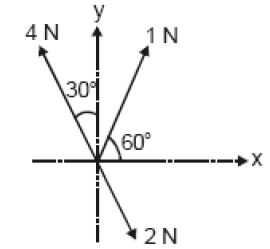A :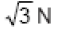B : 0.5N
C : 1.5N
D :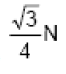•
.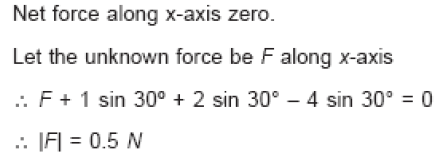•   Question 9

Water falls from a height of 60 m at the rate of 15 kg/s to operate a turbine. The losses due to frictional forces are 10% of energy. How much power is generated by the turbine? (g = 10 m/s2)

A : 7.0 kW
B : 8.1 kW
C : 10.2 kW
D : 12.3 kW

•
.

Solution :
P = 0.9 gH (dm/dt)

•   Question 10

A shell of mass 200 gm is ejected from a gun of mass 4 kg by an explosion that generates 1.05 kJ of energy. The initial velocity of the shell is

A : 120 ms-1
B : 100 ms-1
C : 80 ms-1
D : 40 ms-1

•
.•   Question 11

The ratio of the radii of gyration of a circular disc to that of a circular ring, each of same mass and radius, around their respective axes is

A :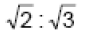B :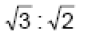C :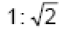D :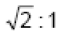•
.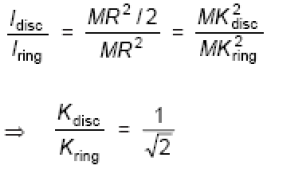•   Question 12

A thin rod of length L and mass M is bent at its midpoint into two halves so that the angle between them is 90o. The moment of inertia of the bent rod about an axis passing through the bending point and perpendicular to the plane defined by the two halves of the rod is

A :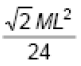B : ML^2/24
C : ML^2/12
D : ML^2/6

•
.

Solution :
Distribution of masses about axis of rotation remain unchanged whether it is straight or bend. I=ML^2/12

•   Question 13

A roller coaster is designed such that riders experience ''weightlessness'' as they go round the top of a hill whose radius of curvature is 20 m. The speed of the car at the top of the hill is between

A : 13 m/s and 14 m/s
B : 14 m/s and 15 m/s
C : 15 ms/ and 16 m/s
D : 16 m/s and 17 m/s

•
.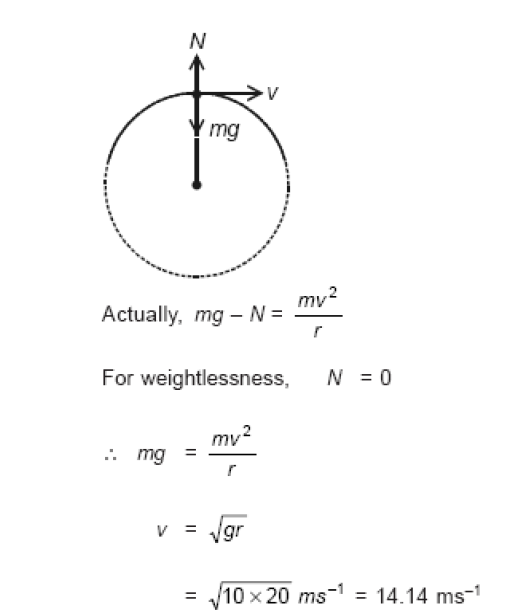•   Question 14

If Q, E and W denote respectively the heat added, change in internal energy and the work done in a closed cycle process, then

A : Q = 0
B : W = 0
C : Q = W = 0
D : E = 0

•
.

Solution :
From the 1st law of thermodynamics, Q = E + W For cyclic processes E=0

•   Question 15

On a new scale of temperature (which is linear) and called the W scale, the freezing and boiling points of water are 39o W and 239o W respectively. What will be the temperature on the new scale, corresponding to a temperature of 39o C on the Celsius scale?

A : 139o W
B : 78o W
C : 117o W
D : 200o W

•
.

Solution :
(39-0)/(100-0)=(x-39)/(239-39) x=117o W

•   Question 16

At 10oC the value of the density of a fixed mass of an ideal gas divided by its pressure is x. At 110oC this ratio is

A : (283/383)x
B : x
D : (10/110)x

•
.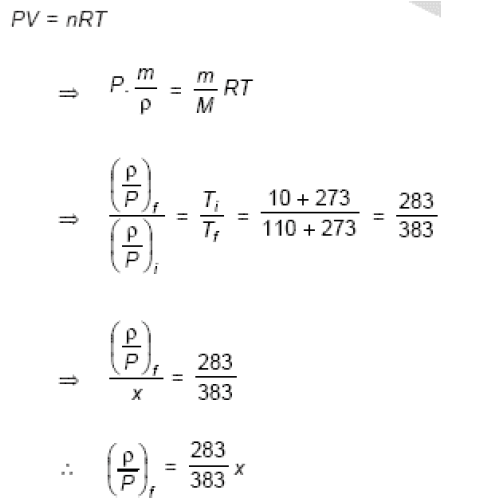•   Question 17

Two Simple Harmonic Motions of angular frequency 100 and 1000 rad s-1 have the same displacement amplitude. The ratio of their maximum accelerations is

A : 1 : 10^4
B : 1 : 10
C : 1 : 10^2
D : 1 : 10^3

•
.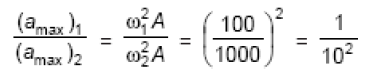•   Question 18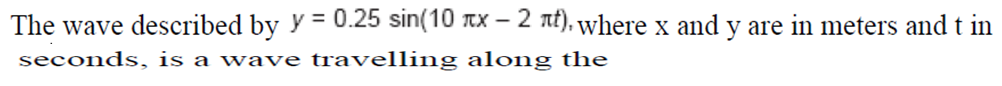A : -ve x direction with amplitude 0.25 m and wavelength = 0.2 m
B : -ve x direction with frequency 1 Hz
C : +ve x direction with frequency pie Hz and wavelength = 0.2 m
D : +ve x direction with frequency 1 Hz and wavelength = 0.2 m

•
.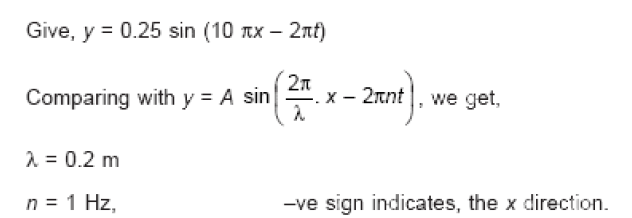•   Question 19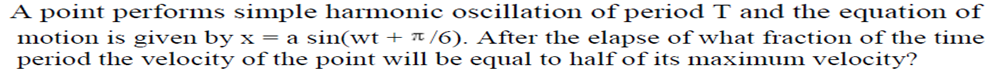A : T/12
B : T/8
C : T/6
D : T/3

•
.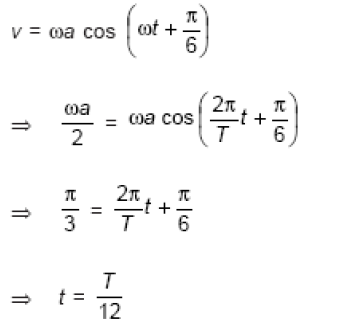•   Question 20

Two points are located at a distance of 10 m and 15 m from the source of oscillation. The period of oscillation is 0.05 sec and the velocity of the wave is 300 m/sec. What is the phase difference between the oscillations of two points?

A :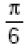B :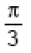C :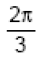D :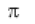•
.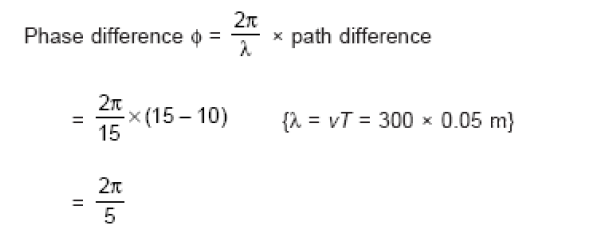•   Question 21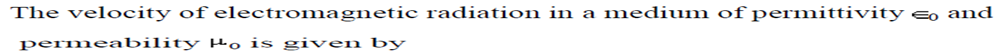A :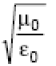B :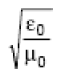C :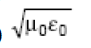D :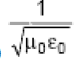•
.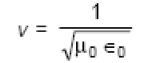•   Question 22

Two periodic waves of intensities I1 and I2 pass through a region at the same time in the same direction. The sum of the maximum and minimum intensities is

A : 2(I1 + I2)
B : I1 + I2
C :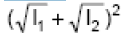D :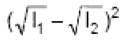•
.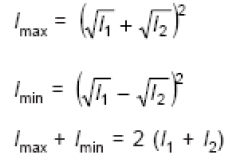•   Question 23

Two thin lenses of focal lengths f1 and f2 are in contact and coaxial. The power of the combinations is

A :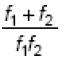B :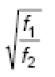C :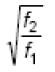D :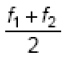•
.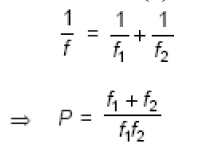•   Question 24

A boy is trying to start a fire by focusing Sunlight on a piece of paper using an equiconvex lens of focal length 10 cm. The diameter of the Sun is 1.39 * 109 m and its mean distance from the earth is 1.5 * 1011 m. What is the diameter of the Sun's image on the paper?

A : 12.4 * 10-4 m
B : 9.2 * 10-4 m
C : 6.5 * 10-4 m
D : 6.5 * 10-5 m

•
.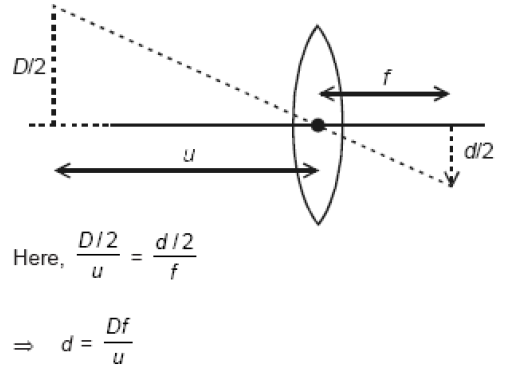•   Question 25

The energy required to charge a parallel plate condenser of plate separation d and plate area of cross-section A such that the uniform electric field between the plates is E, is

A :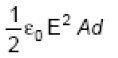B :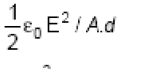C :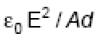D :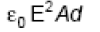•
.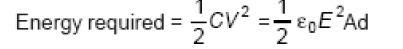•   Question 26

A thin conducting ring of radius R is given a charge +Q. The electric field at the centre O of the ring due to the charge on the part AKB of the ring is E. The electric field at the centre due to the charge on the part ACDB of the ring is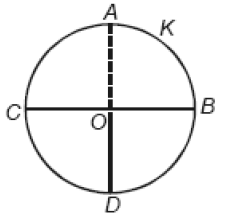A : 3 E along OK
B : 3 E along KO
C : E along OK
D : E along KO

•
.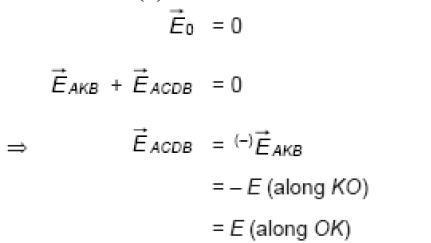•   Question 27

The electric potential at a point in free space due to a charge Q coulomb is Q � 1011 volts. The electric field at that point is

A :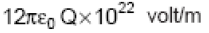B :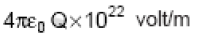C :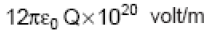D :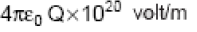•
.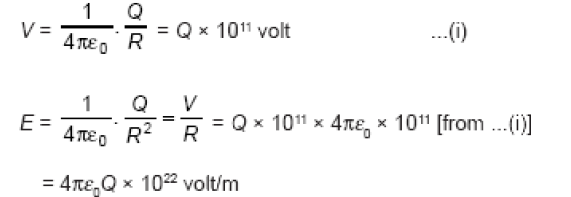•   Question 28

A cell can be balanced against 110 cm and 100 cm of potentiometer wire, respectively with and without being short circuited through a resistance of 10ohm . Its internal resistance is

A : 0
B : 1.0ohm
C : 0.5ohm
D : 2.0ohm

•
.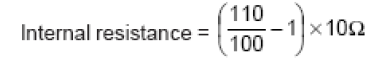•   Question 29

wire of a certain material is stretched slowly by ten per cent. It new resistance and specific resistance become respectively

A : 1.1 times, 1.1 times
B : 1.2 times, 1.1 times
C : 1.21 times, same
D : Both remain the same

•
.

Solution :
R2/R1= (I2/I1)2=1.21

•   Question 30

In the circuit shown, the current through the 4ohm resistor is 1 amp when the points P and M are connected to a d.c. voltage source. The potential difference between the points M and N is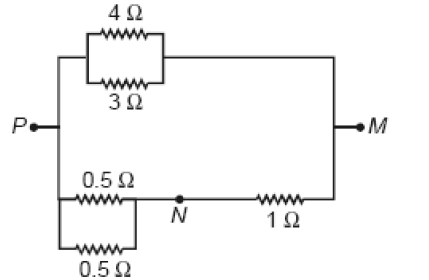A : 3.2volt
B : 1.5volt
C : 1.0volt
D : 0.5volt

•
.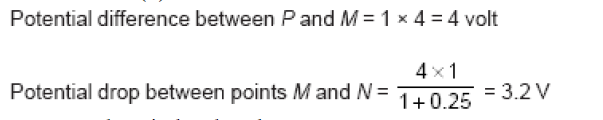•   Question 31

An electric kettle takes 4A current at 220 V. How much time will it take to boil 1 kg of water from temperature 20 deg C? The temperature of boiling water is 100 deg C.

A : 4.2 min
B : 6.3 min
C : 8.4 min
D : 12.6 min

•
.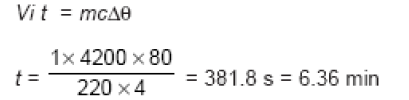•   Question 32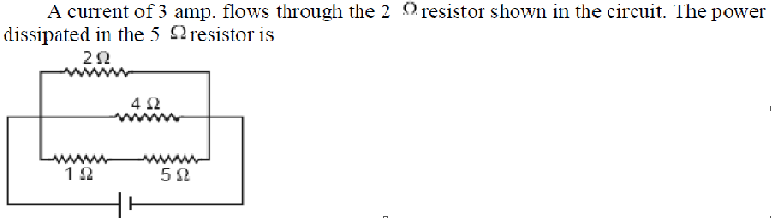A : 5watt
B : 4watt
C : 2watt
D : 1watt

•
.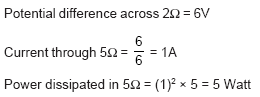•   Question 33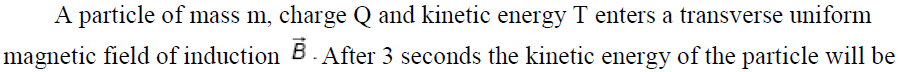A : 4T
B : 3T
C : 2T
D : T

•
.

Solution :
Work done by magnetic field on charge particle is zero. Therefore its kinetic energy will be same i.e. T

•   Question 34

A closed loop PQRS carrying a current is placed in a uniform magnetic field. If the magnetic forces on segments PS, SR and RQ are F1, F2 and F3 respectively and are in the plane of the paper and along the directions shown, the force on the segment QP is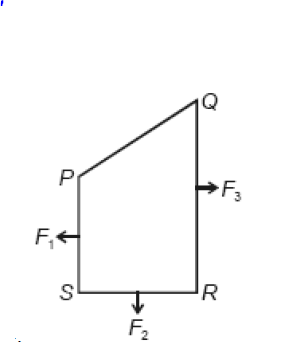A : F3 - F1 + F2
B : F3 - F1 - F2
C :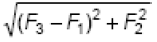D :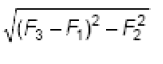•
.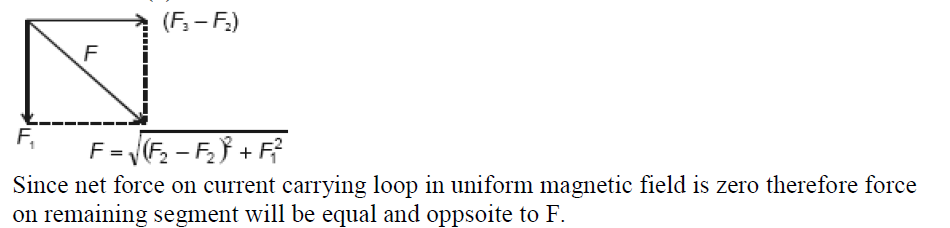•   Question 35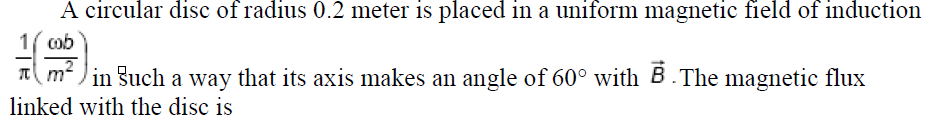A : 0.01wb
B : 0.02wb
C : 0.06wb
D : 0.08wb

•
.